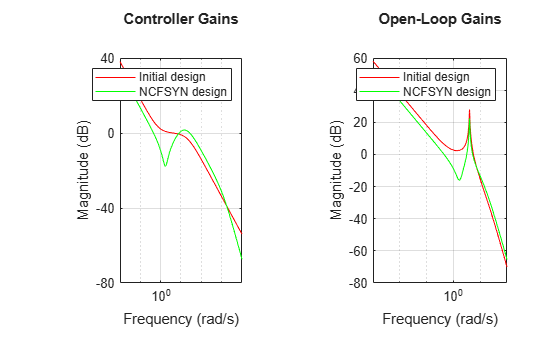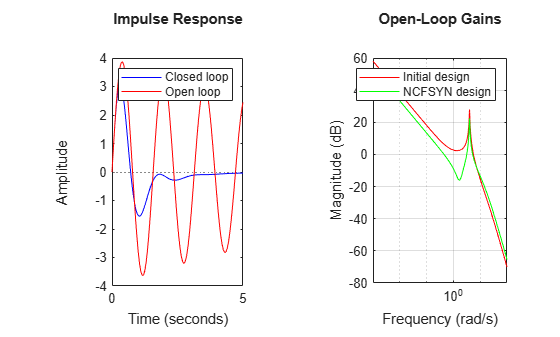# Improving Stability While Preserving Open-Loop Characteristics

This example shows how to use Robust Control Toolbox™ function ncfsyn to improve the stability robustness of a closed-loop system while approximately maintaining the high-gain and low-gain characteristics of the open-loop response.

### Plant Model

The plant model is a lightly-damped, second-order systemA Bode plot clearly shows the resonant peak.

P = tf(16,[1 0.16 16]); bode(P)### Closed-Loop Objectives and Initial Controller Design

The closed-loop system should

• Have integral action, and a sensitivity bandwidth of at least 0.5 rad/sec

• Have gain-crossover frequencies no larger than 7

We can translate these requirements into a desired shape for the open-loop gain and seek a controller that enforces this shape. For example, a controller Kprop consisting of a PI term in series with a high-frequency lag component achieves the desired loop shape:

K_PI = pid(1,0.8); K_rolloff = tf(1,[1/20 1]); Kprop = K_PI*K_rolloff; bodemag(P*Kprop); gridThe desired high-frequency rolloff, integral action, and location of the gain-crossover frequency are all evident from the graph. Unfortunately, this controller does not stabilize the closed-loop system, as evidenced by the Stable field from the allmargin calculation.

allmargin(P*Kprop) 
ans = struct with fields: GainMargin: 0.1096 GMFrequency: 4.1967 PhaseMargin: -20.4811 PMFrequency: 5.6155 DelayMargin: 1.0552 DMFrequency: 5.6155 Stable: 0 

### Closed-loop Stability and Margin Improvement Using ncfsyn

The command ncfsyn can be used to obtain closed-loop stability and improved stability margins without significant degradation in the large (>>1) and small (<<1) gain regions of the open-loop response. A value of Gamma (the 3rd output argument) less than 3 indicates success (modest gain degradation along with acceptable robustness margins). Note that ncfsyn assumes positive feedback so you must flip the sign of Kprop. The new controller, K, stabilizes the nominal dynamics, and appears to have decent robustness margins, at least when measured in the classical sense.

[negK,~,Gamma] = ncfsyn(P,-Kprop); K = -negK; % flip sign back Gamma allmargin(P*K) 
Gamma = 1.9883 ans = struct with fields: GainMargin: [6.3299 11.1423] GMFrequency: [1.6110 15.1667] PhaseMargin: [80.0276 -99.6641 63.7989] PMFrequency: [0.4472 3.1460 5.2319] DelayMargin: [3.1236 1.4443 0.2128] DMFrequency: [0.4472 3.1460 5.2319] Stable: 1 

As expected, the closed-loop is stable, and with Gamma less than 2, the gain and phase margins are adequate by rule-of-thumb standards.

### Bode Magnitude of Controllers

Gamma gives an indication of the gain degradation (in going from Kprop to K) we should expect, both the in the large and small loop-gain regions. With Gamma approximately 2, we expect only about 6dB (20*log10(Gamma)) gain reduction in the large loop-gain regions, and no more than 6dB gain increase in the small loop-gain regions. Bode magnitude plots confirm this.

subplot(1,2,1) bodemag(Kprop,'r',K,'g'); grid legend('Proposed Controller, Kprop','Redesigned Controller, K') title('Controller Gains') subplot(1,2,2) bodemag(P*Kprop,'r',P*K,'g'); grid legend('P*Kprop','P*K') title('Open-Loop Gains')Figure 1: Controller and Open-Loop gains

### Closed-Loop Response to Impulse at the Plant Input

An impulsive disturbance at the plant input is damped out effectively in a few seconds. For comparison, the uncompensated plant response is also shown.

TF = 4; subplot(1,2,1) impulse(feedback(P,K),'b',P,'r',TF); legend('Closed-Loop','Open-Loop') title('Plant response, y, to impulse at plant input') subplot(1,2,2); impulse(-feedback(K*P,1),'b',TF) legend('Closed-Loop Control action, u')Figure 2: Controller and Open-Loop gains

### Sensitivity and Complementary Sensitivity Functions

Finally, the closed-loop Sensitivity and Complementary Sensitivity functions show the desired sensitivity reduction and high-frequency noise attenuation expressed in the closed-loop performance objectives.

S = feedback(1,P*K); T = 1-S; clf bodemag(S,T,{1e-2,1e2}); grid### Conclusions

In this example, the function ncfsyn is used to adjust a proposed controller in order to achieve closed-loop stability, while attempting to maintain the general large and small loop-gain characteristics of the open-loop function PK.

##### SupportGet trial now